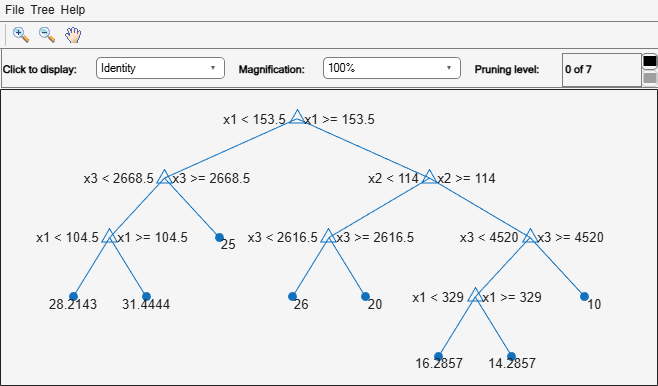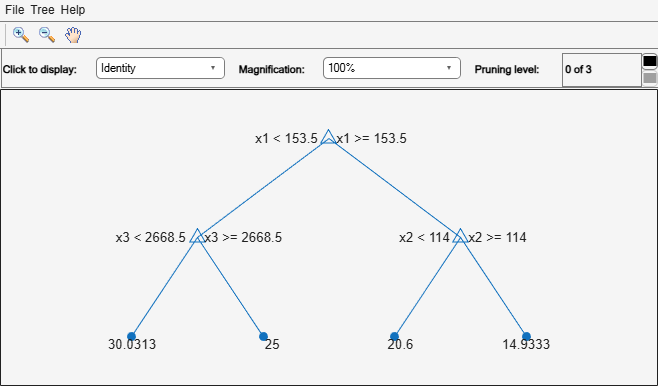resubLoss

Class: RegressionTree

Regression error by resubstitution

Syntax

L = resubLoss(tree)
L = resubLoss(tree,Name,Value)
L = resubLoss(tree,'Subtrees',subtreevector)
[L,se] = resubLoss(tree,'Subtrees',subtreevector)
[L,se,NLeaf] = resubLoss(tree,'Subtrees',subtreevector)
[L,se,NLeaf,bestlevel] = resubLoss(tree,'Subtrees',subtreevector)
[L,...] = resubLoss(tree,'Subtrees',subtreevector,Name,Value)

Description

L = resubLoss(tree) returns the resubstitution loss, meaning the loss computed for the data that fitrtree used to create tree.

L = resubLoss(tree,Name,Value) returns the loss with additional options specified by one or more Name,Value pair arguments. You can specify several name-value pair arguments in any order as Name1,Value1,…,NameN,ValueN.

L = resubLoss(tree,'Subtrees',subtreevector) returns a vector of mean squared errors for the trees in the pruning sequence subtreevector.

[L,se] = resubLoss(tree,'Subtrees',subtreevector) returns the vector of standard errors of the classification errors.

[L,se,NLeaf] = resubLoss(tree,'Subtrees',subtreevector) returns the vector of numbers of leaf nodes in the trees of the pruning sequence.

[L,se,NLeaf,bestlevel] = resubLoss(tree,'Subtrees',subtreevector) returns the best pruning level as defined in the TreeSize name-value pair. By default, bestlevel is the pruning level that gives loss within one standard deviation of minimal loss.

[L,...] = resubLoss(tree,'Subtrees',subtreevector,Name,Value) returns loss statistics with additional options specified by one or more Name,Value pair arguments. You can specify several name-value pair arguments in any order as Name1,Value1,…,NameN,ValueN.

Input Arguments

expand all

A regression tree (RegressionTree model object) constructed using fitrtree.

Name-Value Arguments

Specify optional comma-separated pairs of Name,Value arguments. Name is the argument name and Value is the corresponding value. Name must appear inside quotes. You can specify several name and value pair arguments in any order as Name1,Value1,...,NameN,ValueN.

Loss function, specified as a function handle or 'mse' meaning mean squared error.

You can write your own loss function in the syntax described in Loss Functions.

Data Types: char | string | function_handle

Name,Value arguments associated with pruning subtrees:

Pruning level, specified as the comma-separated pair consisting of 'Subtrees' and a vector of nonnegative integers in ascending order or 'all'.

If you specify a vector, then all elements must be at least 0 and at most max(tree.PruneList). 0 indicates the full, unpruned tree and max(tree.PruneList) indicates the completely pruned tree (i.e., just the root node).

If you specify 'all', then resubLoss operates on all subtrees (i.e., the entire pruning sequence). This specification is equivalent to using 0:max(tree.PruneList).

resubLoss prunes tree to each level indicated in Subtrees, and then estimates the corresponding output arguments. The size of Subtrees determines the size of some output arguments.

To invoke Subtrees, the properties PruneList and PruneAlpha of tree must be nonempty. In other words, grow tree by setting 'Prune','on', or by pruning tree using prune.

Example: 'Subtrees','all'

Data Types: single | double | char | string

Tree size, specified as one of the following:

• 'se'loss returns the highest pruning level with loss within one standard deviation of the minimum (L + se, where L and se relate to the smallest value in Subtrees).

• 'min'loss returns the element of Subtrees with smallest loss, usually the smallest element of Subtrees.

Output Arguments

expand all

Regression loss (mean squared error), a vector the length of Subtrees. The meaning of the error depends on the values in Weights and LossFun.

Standard error of loss, a vector the length of Subtrees.

Number of leaves (terminal nodes) in the pruned subtrees, a vector the length of Subtrees.

A scalar whose value depends on TreeSize:

• TreeSize = 'se'loss returns the highest pruning level with loss within one standard deviation of the minimum (L + se, where L and se relate to the smallest value in Subtrees).

• TreeSize = 'min'loss returns the element of Subtrees with smallest loss, usually the smallest element of Subtrees.

Examples

expand all

Load the carsmall data set. Consider Displacement, Horsepower, and Weight as predictors of the response MPG.

X = [Displacement Horsepower Weight];

Grow a regression tree using all observations.

Mdl = fitrtree(X,MPG);

Compute the resubstitution MSE.

resubLoss(Mdl)
ans = 4.8952

Unpruned decision trees tend to overfit. One way to balance model complexity and out-of-sample performance is to prune a tree (or restrict its growth) so that in-sample and out-of-sample performance are satisfactory.

Load the carsmall data set. Consider Displacement, Horsepower, and Weight as predictors of the response MPG.

X = [Displacement Horsepower Weight];
Y = MPG;

Partition the data into training (50%) and validation (50%) sets.

n = size(X,1);
rng(1) % For reproducibility
idxTrn = false(n,1);
idxTrn(randsample(n,round(0.5*n))) = true; % Training set logical indices
idxVal = idxTrn == false;                  % Validation set logical indices

Grow a regression tree using the training set.

Mdl = fitrtree(X(idxTrn,:),Y(idxTrn));

View the regression tree.

view(Mdl,'Mode','graph');The regression tree has seven pruning levels. Level 0 is the full, unpruned tree (as displayed). Level 7 is just the root node (i.e., no splits).

Examine the training sample MSE for each subtree (or pruning level) excluding the highest level.

m = max(Mdl.PruneList) - 1;
trnLoss = resubLoss(Mdl,'SubTrees',0:m)
trnLoss = 7×1

5.9789
6.2768
6.8316
7.5209
8.3951
10.7452
14.8445

• The MSE for the full, unpruned tree is about 6 units.

• The MSE for the tree pruned to level 1 is about 6.3 units.

• The MSE for the tree pruned to level 6 (i.e., a stump) is about 14.8 units.

Examine the validation sample MSE at each level excluding the highest level.

valLoss = loss(Mdl,X(idxVal,:),Y(idxVal),'SubTrees',0:m)
valLoss = 7×1

32.1205
31.5035
32.0541
30.8183
26.3535
30.0137
38.4695

• The MSE for the full, unpruned tree (level 0) is about 32.1 units.

• The MSE for the tree pruned to level 4 is about 26.4 units.

• The MSE for the tree pruned to level 5 is about 30.0 units.

• The MSE for the tree pruned to level 6 (i.e., a stump) is about 38.5 units.

To balance model complexity and out-of-sample performance, consider pruning Mdl to level 4.

pruneMdl = prune(Mdl,'Level',4);
view(pruneMdl,'Mode','graph')